https://doi.org/10.5194/tc-13-1283-2019
https://doi.org/10.5194/tc-13-1283-2019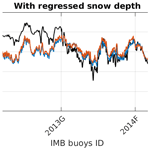# Estimating the snow depth, the snow–ice interface temperature, and the effective temperature of Arctic sea ice using Advanced Microwave Scanning Radiometer 2 and ice mass balance buoy data

Lise Kilic, Rasmus Tage Tonboe, Catherine Prigent, and Georg Heygster
Abstract

Mapping sea ice concentration (SIC) and understanding sea ice properties and variability is important, especially today with the recent Arctic sea ice decline. Moreover, accurate estimation of the sea ice effective temperature (Teff) at 50 GHz is needed for atmospheric sounding applications over sea ice and for noise reduction in SIC estimates. At low microwave frequencies, the sensitivity to the atmosphere is low, and it is possible to derive sea ice parameters due to the penetration of microwaves in the snow and ice layers. In this study, we propose simple algorithms to derive the snow depth, the snow–ice interface temperature (TSnow−Ice) and the Teff of Arctic sea ice from microwave brightness temperatures (TBs). This is achieved using the Round Robin Data Package of the ESA sea ice CCI project, which contains TBs from the Advanced Microwave Scanning Radiometer 2 (AMSR2) collocated with measurements from ice mass balance buoys (IMBs) and the NASA Operation Ice Bridge (OIB) airborne campaigns over the Arctic sea ice. The snow depth over sea ice is estimated with an error of 5.1 cm, using a multilinear regression with the TBs at 6, 18, and 36 V. The TSnow−Ice is retrieved using a linear regression as a function of the snow depth and the TBs at 10 or 6 V. The root mean square errors (RMSEs) obtained are 2.87 and 2.90 K respectively, with 10 and 6 V TBs. The Teff at microwave frequencies between 6 and 89 GHz is expressed as a function of TSnow−Ice using data from a thermodynamical model combined with the Microwave Emission Model of Layered Snowpacks. Teff is estimated from the TSnow−Ice with a RMSE of less than 1 K.

Share
Dates
1 Introduction

In situ observations of the variables controlling the sea ice energy and momentum balance in polar regions are scarce. One way to overcome this observational gap is to use satellites for measuring sea ice properties. The objective of this study is to estimate key sea ice variables from satellite remote sensing to improve current sea ice models and prediction, sea ice concentration (SIC) mapping in the EUMETSAT Ocean and Sea Ice Satellite Application Facility (OSISAF) project, and polar atmospheric sounding applications.

Sea ice thermodynamics is controlled by the regional heat budget . In general, sea ice is covered by snow, which can reach a mean thickness of up to ∼50 cm in the Arctic . Snow on sea ice strongly affects the sea ice energy and radiation balance, with its high insulation of heat and reflectivity of solar radiation. Snow is a poor conductor of heat: it insulates the sea ice and reduces the winter ice growth . In summer, its high albedo reduces the sea ice melting rate. The high albedo of snow on sea ice compared to open-water albedo plays an important role in the sea ice albedo feedback mechanism and Arctic amplification (Hall2004). suggest that the recent sea ice growth has been effectively limited by the increase in snow depth on thin ice during winter. Current sea ice models include snow schemes (e.g. ), with the snow depth and temperature gradient in the snow pack modulating the sea ice growth and melt. Improved estimates of snow depth (Ds), as well as snow–ice interface temperature (TSnow−Ice) from satellite observations would provide valuable information on the vertical thermodynamics in the snow and ice to improve current sea ice models and therefore the prediction of sea ice growth.

Here we propose using a simple algorithm to retrieve Ds and TSnow−Ice from passive microwave observations from the Advanced Microwave Scanning Radiometer 2 (AMSR2), based on a large data set of collocated in situ and satellite observations. An extensive Round Robin Data Package (RRDP) (, https://figshare.com/articles/Reference_dataset_for_sea_ice_concentration/6626549, last access: 15 January 2019) has been developed during the European Space Agency (ESA) sea ice Climate Change Initiative (CCI) project and the SPICES (Space-borne observations for detecting and forecasting sea ice cover extremes) project (http://www.seaice.dk/ecv2/rrdb-v1.1/, last access: 15 June 2017). It contains in situ data from the ice mass balance buoys (IMBs), and the Operation Ice Bridge (OIB) airborne campaigns collocated with AMSR2 brightness temperature measurements between 6 and 89 GHz.

Algorithms already exist to retrieve the snow depth from microwave observations. and use the spectral gradient ratio of the 19 and 37 GHz (GR37/19) in vertical polarization to deduce the snow depth over sea ice. This method has been developed for dry snow on first-year ice (FYI) in Antarctica, and it is applicable only to this ice type. Sea ice emissivity depends on the ice type. At frequencies ≥18 GHz, the ice emissivity is higher for FYI than for multi-year ice (MYI) . The difference of emissivity between the 19 and 37 GHz can be used to retrieve the snow depth or the sea ice type. Therefore, the snow depth algorithms which use this gradient ratio (GR37/19) are strongly dependent on the ice type. Improvements by have been suggested by and . More recently, revisit the methodology for the Arctic region, using a new gradient ratio between 7 and 19 GHz (GR19/7) to derive snow depths over both FYI and MYI. For their study, they use the snow depths of OIB campaigns obtained in March and April. With the help of the RRDP, we will extend the methodology to the full winter (from 1 December to 1 April) for the Arctic region using the IMB snow depth data.

showed from radiative transfer simulations that there is a high linear correlation between the TSnow−Ice and the passive microwave observations at 6 GHz. Preliminary results from evidenced the possibility of deriving the temperature of sea ice from passive microwave observations using simple regression models. This work will be extended here to estimate TSnow−Ice over Arctic sea ice.

Passive microwave satellite observations between 50 and 60 GHz are extensively used to provide the atmospheric temperature profiles in Numerical Weather Prediction (NWP) centres, with instruments such as the Advanced Microwave Sounding Unit-A (AMSU-A) or the Advanced Technology Microwave Sounder (ATMS). For an accurate estimation of the temperature profile in the lower atmosphere, quantifying the surface contribution is required. The surface contribution, i.e. the surface brightness temperature (TB), depends on the frequency, and it is the product of a surface effective emissivity (eeff) and a surface effective temperature (Teff):

$\begin{array}{}\text{(1)}& \text{TB}={e}_{\mathrm{eff}}\cdot {T}_{\mathrm{eff}}.\end{array}$

Teff is defined as the integrated temperature over a layer corresponding to the penetration depth at the given frequency: the larger the wavelength, the deeper the penetration into the medium. In the same way, eeff represents the integrated emissivity over a layer corresponding to the penetration depth. It depends on the frequency, the incidence angle, and the sub-surface extinction and reflections between snow and sea ice layers (Tonboe2010). Therefore, estimating the surface contribution is particularly complicated over sea ice due to the layering and the vertical structure of the snowpack, which affect the microwave emission processes , and to the large spatial and temporal variability of sea ice and snow cover . The understanding of the relationship between Teff and the physical temperature profile is complicated, especially at microwave frequencies ≥18 GHz, when scattering occurs, but it has been shown that from 6 to 50 GHz there is a high correlation between the Teff and the TSnow−Ice . With TSnow−Ice estimated from the AMSR2 observations, we will deduce the sea ice Teff at AMSR2 frequencies between 6 and 89 GHz, using linear regression.

Section 2 describes the data set and the methodology used in this study. The snow depth retrieval is presented in Sect. 3. Section 4 reports on the TSnow−Ice retrieval. Finally, microwave sea ice Teff at 50 GHz is derived for application to temperature atmospheric sounding (Sect. 5). Section 6 discusses the snow depth and the TSnow−Ice retrieval results over a winter in Arctic. Section 7 concludes this study.

2 Material and methods

## 2.1 The database of collocated satellite observations and in situ measurements

The RRDP from the ESA sea ice CCI project is an openly available data set (, https://figshare.com/articles/Reference_dataset_for_sea_ice_concentration/6626549, last access: 15 January 2019). It contains an extensive collection of collocated satellite microwave radiometer data with in situ buoy or airborne campaign measurements and other geophysical parameters, with relevance for computing and understanding the variability of the microwave observations over sea ice. It covers areas with 0 % and 100 % of SIC and different sea ice types (thin ice, first-year ice, multiyear ice), for all seasons including summer melt. In our study, we will focus on Arctic sea ice during winter in regions with 100 % sea ice cover. Two different data sets from the RRDP are used: AMSR2 brightness temperatures (TBs) collocated with IMB measurements and AMSR2 TBs collocated with OIB airborne campaign measurements.

AMSR2 is a passive microwave radiometer on board the JAXA GCOM-W1 satellite (launched on 18 May 2012). AMSR2 has 14 channels at 6.9, 7.3, 10.65, 18.7, 23.8, 36.5, and 89 GHz for both vertical and horizontal polarizations and it observes at 55 of incidence angle. In the RRDP, the spatial resolution of each channel is resampled by JAXA to the 6.9 GHz resolution (32×62 km) (see AMSR2 L1R products, ) before collocation with buoy or airborne campaign measurements (RRDP report, ).

IMBs are installed by the Cold Regions Research and Engineering Laboratory (CRREL) to measure the ice mass balance of the Arctic sea ice cover . Buoy components include acoustic sounders and a string of thermistors. The thermistor string extends from the air, through the snow cover and sea ice, into the water and has temperature sensors located every 10 cm along the string. It measures the physical temperature with an accuracy of 0.1 K. There are two acoustic sounders located above the snow surface and below the sea ice. The acoustic sounders measure the position of snow and ice surfaces (top and bottom) with a precision of 5 mm, from which the snow depth is computed. The buoys also include instruments that measure air temperature, barometric air pressure, and GPS geographical position . Several IMBs are deployed by the CRREL at different locations and times during the year. We only use Arctic buoy data recorded during winter (1 December to 1 April) to avoid cases where ice starts to melt. The IMBs available for this study are all located on MYI, with an ice thickness ≥1 m. A summary of buoy information corresponding to these criteria is given in Table 1 and the IMB locations are shown in Fig. 1. IMB measurements collocated with AMSR2 TBs used in this study totalize 2845 observations.

Table 1List of the IMBs used in this study, with the mean snow depth (column 5) and the mean ice thickness (column 6) computed over the duration of the measurements (column 2).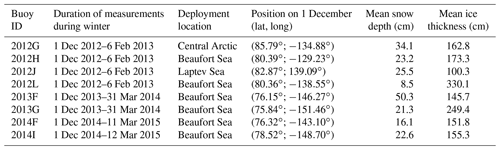For snow depth retrieval, we also used data from the OIB airborne campaign. The NASA OIB project has collected ice and snow depth data in the Arctic during annual flight campaigns (March–May) since 2009. The data are especially valuable in this context, since they contain snow depth information from the snow radar on board the aircraft, not only from single points but continuously along the flight path. The vertical resolution of the OIB snow radar is 3 cm, and the uncertainty on the snow depth is around 6 cm compared with in situ measurements . Recent studies evidence larger errors on OIB snow depth with issues to detect snow depth under 8 cm . These different limitations are summarized in . In the RRDP, the snow depth data from OIB snow radar are averaged into 50 km sections to be collocated with AMSR2 observations. For our study we use the OIB data from the 2013 campaign. It totalizes 408 observations over 8 d in March and April and covers FYI and MYI areas. Figure 1 summarizes the locations of IMBs and OIB campaigns over the Arctic ocean.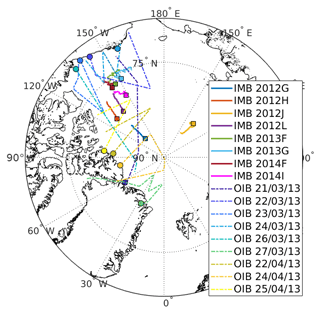Figure 1Ice mass balance buoy and Operation Ice Bridge (OIB) flight locations over Arctic sea ice. Squares indicate the position of IMBs on 1 December and circles indicate the starting points of the OIB campaigns.

It is important to note that there are discrepancies due to the scale when comparing point measurements from buoys with the spatially averaged data from satellites or aircrafts .

## 2.2 The database of simulated effective temperature and brightness temperature from sea ice properties

For the estimation of Teff, we use a microwave emission model coupled with a thermodynamic model. The emission model uses the temperature, density, snow crystal and brine inclusion size, salinity, and snow or ice type to estimate the microwave emissivity, the Teff, and the TB of sea ice. It is coupled with a thermodynamic model in order to provide realistic microphysical inputs. The thermodynamic model for snow and sea ice is forced with ECMWF ERA40 meteorological data input: surface air pressure, 2 m air temperature, wind speed, incoming shortwave and longwave radiation, relative humidity, and accumulated precipitation. It computes a centimetre-scale profile of the parameters used as inputs to the emission model. The emission model used here is a sea ice version of the Microwave Emission Model of Layered Snowpacks (MEMLS) described in . The simulations were part of an earlier version of the RRDP and the simulation methodology is described in . This MEMLS simulation uses, among its inputs, the snow depth and the TSnow−Ice and computes Teffs and TBs at different frequencies (from 1.4 to 183 GHz). The data set contains 1100 cases and is called the MEMLS-simulated data set in the following.

## 2.3 Methodology

In this study, we propose simple algorithms, using multilinear regressions, to derive the snow depth, the TSnow−Ice, and the Teff of sea ice from AMSR2 TBs.

The measurements from the IMB 2012G, 2012H, 2012J, and 2012L, collocated with AMSR2 TBs, are used as the training data set for the different regressions to retrieve snow depth and TSnow−Ice. These buoys have been selected because they are located in different regions across the Arctic and show a large range of snow depths. The measurements from IMB 2013F, 2013G, 2014F, and 2014I, which are all located in the Beaufort Sea, are used as the testing data set.

First, the IMB snow depth is expressed as a function of the AMSR2 TBs using a multilinear regression (see Sect. 3.1). The OIB data are used for the forward selection and the IMB training data set is used to perform the regression. Second, the TSnow−Ice is expressed as a function of TBs and snow depth, using linear regressions. An automated method is developed that detects the position of the snow–ice interface on the vertical temperature profile measured by the IMB thermistor string (see Sect. 4.1). Then, the IMB training data set is used to perform the regressions (see Sect. 4.3). For this part there are two consecutive regressions: the first one is done between the centred (the average was subtracted) TSnow−Ice and TBs; the second one is done between the TSnow−Ice corrected for the TB dependence and the snow depth. Third, the sea ice Teff at different microwave frequencies is expressed as a function of the TSnow−Ice (see Sect. 5.2). This final step uses the simulations from a thermodynamical model and MEMLS to derive linear regression equations for the Teff at frequencies between 6 and 89 GHz. The Teff at 50 GHz is of special interest for atmospheric sounding applications.

3 Snow depth estimation

## 3.1 Multilinear regression to retrieve the snow depth

A forward selection method is used to choose the best AMSR2 channels to retrieve snow depth. It is a statistical method to determine the best-predictor combinations (here, AMSR2 TBs) to retrieve a variable (here, snow depth). We use the stepwise regression . It is a sequential predictor selection technique: at each step statistic tests are computed, and the predictors included in the model are adjusted. Our training data set for this forward selection is the OIB snow depth from the 2013 campaign included in the RRDP. OIB data are chosen for forward selection because the data cover a large area with a wide range of snow depths. In addition, the scale of the averaged OIB data is closer to satellite footprint than buoy measurements, increasing the consistency with the satellite observations. Forward selection tests have also been done with the IMB training data set, but the results were not satisfactory. We find that the best channel combination for snow depth retrieval is the combination of the three channels at 6.9, 18.7, and 36.5 GHz in vertical polarization (6, 18, and 36 V).

Then, a multilinear regression is conducted using the IMB training data set (buoys G, H, J, L in 2012 collocated with AMSR2 TBs). The snow depth is given as a linear combination of the TBs at 6, 18, and 36 V:

$\begin{array}{ll}& {D}_{\mathrm{s}}=\mathrm{1.7701}+\mathrm{0.0175}\cdot {\text{TB}}_{\mathrm{6}\phantom{\rule{0.125em}{0ex}}\mathrm{V}}-\mathrm{0.0280}\cdot {\text{TB}}_{\mathrm{18}\phantom{\rule{0.125em}{0ex}}\mathrm{V}}\\ \text{(2)}& & \phantom{\rule{0.25em}{0ex}}\phantom{\rule{0.25em}{0ex}}\phantom{\rule{0.25em}{0ex}}+\mathrm{0.0041}\cdot {\text{TB}}_{\mathrm{36}\phantom{\rule{0.125em}{0ex}}\mathrm{V}},\end{array}$

with Ds the snow depth expressed in metres and TB in kelvin. This model was trained with snow depths between 5 and 40 cm.

The forward selection has also been tested by constraining the number of predictors to 2 and 4. The combinations obtained are 18 and 36 V for two channels and 6, 18, 36, and 89 V for four channels. Then, the multilinear regression has been performed using these combinations of two or four channels. The results show that the three-channel combination is the best in terms of RMSE and correlation compared to the two- or four-channel combination (see Sect. 3.2).

## 3.2 Results of the snow depth retrieval

Figure 2 shows the comparison between the observed snow depth measured by the acoustic sounder of IMB and the regressed snow depth computed from AMSR2 TBs with Eq. (2). The RMSE between the IMB snow depth observations and our snow depth regression is 12.0 cm and the correlation coefficient is 0.66, using the IMBs 2013F, 2013G, 2014F, and 2014I (which are not in the training data set). The buoy 2013F observes a large snow depth (> 40 cm), which is outside the bounds of our snow depth model. Tests are conducted to improve the estimation, including the 2013F buoy in the training data set, with equal numbers of observations for different ranges of snow depths: it does not improve the results. Our model obtained the same snow depth estimation between buoys 2013G and 2013F. It is consistent because these buoys are spatially very close. Therefore, we suspect that the 2013F buoy is located nearby a ridge or hummock, where the local snow depth is large but not detectable at the satellite footprint scale. Without including the buoy 2013F in the computation, the RMSE for our snow depth model is 5.1 cm and the correlation coefficient is 0.61.

We also compare the snow depth retrievals with the measurements of the 2013 OIB campaigns (see Fig. 3) with the ice type computed from the gradient ratio between 19 and 37 GHz . Our snow depth regression (Eq. 2) RMSE is 6.26 cm and the correlation coefficient with OIB observations is 0.87. Note that the uncertainties on OIB data for the 2013 campaigns are between 2 and 22 cm with a mean standard deviation (SD) of 11 cm (OIB snow depth Dsnow provided in the RRDP). Looking at Fig. 3, our snow depth regression is applicable to both ice types. The RMSEs computed for MYI and FYI are 7.2 and 3.9 cm, and the correlations are 0.71 and 0.03. The RMSE is smaller for FYI because the snow depth variability of FYI is also smaller. The low correlation obtained for FYI can come from the limited number of observations and because the snow depth variability observed is within the signal noise.

Spatial scales are different when comparing satellite measurements or airborne campaign measurements with buoy measurements. Discrepancies can appear due to the spatial variability of the snow depth. It can explain that the correlation is higher when comparing snow depth estimated from AMSR2 TBs with the snow depth observed from OIB radar. It is also important to note that the OIB campaign data are from late winter to beginning of spring (March to April), while IMB measurements are from winter (December to March). With the snow depth regression being developed on IMB measurements, this small change in season can contribute to the larger RMSE observed with OIB data.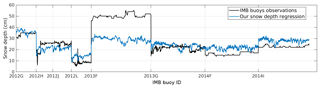Figure 2Time series of the comparison between snow depths from IMB observations and our multilinear regression (Eq. 2). The beginning of the measurements with a new IMB is indicated on the x axis.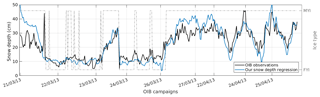Figure 3Time series of the comparison between snow depths (left y axis) from OIB observations and our multilinear regression (Eq. 2). The beginning of the measurements with a new OIB campaign is indicated on the x axis. For each measurement, the ice type is indicated with a dashed grey line (right y axis).

4 Snow–ice interface temperature estimation

## 4.1 Automatic interface position detection

During winter, the air temperature is very cold, meaning that the snow surface temperature is cold compared to ice and water temperatures. Through sea ice, the temperature profile is piecewise linear and temperature increases with depth (see Fig. 4). In the air, the temperature gradient is small because of turbulent mixing. In the snow, the temperature gradient is larger due to the thermal properties of snow. Therefore, air–snow and snow–ice interface positions can be detected by changes in the temperature gradient. At the air–snow interface, the second derivative of the temperature profile reaches a maximum. At the snow–ice interface, the temperature gradient being lower in the ice than in the snow, the second derivative of the temperature profile reaches a minimum. Using these properties of the sea ice temperature profile, an automated method is implemented to detect the air–snow and the snow–ice interface positions in the temperature profile measured by the buoy thermistor string.

Figure 4 shows an averaged temperature profile through sea ice during winter, with the air–snow and snow–ice interface positions detected with our automated method. This method performs best during winter when the air is cold. It may not be applicable if the snow depth is lower than the vertical resolution of the thermistor string (10 cm) or if sea ice starts to melt and the temperature profile develops gradually toward an isothermal state. The method selects the thermistor which is located the closest to the interface. Note that the real interface position can be located between two thermistors. Therefore, the shift between the real interface position and the thermistor the closest to the interface can be up to 5 cm. This can introduce uncertainties in our TSnow−Ice regression.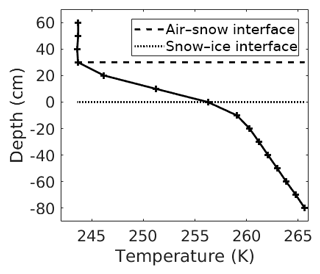Figure 4Averaged temperature profile (from December to February) measured by the IMB 2012G, with air–snow and snow–ice interface levels detected with our automated method.

## 4.2 Correlation between the brightness temperature and the snow–ice interface temperature

During winter, the vertical position of the snow–ice interface is fixed with respect to the buoy thermistor string. The thermistor string is frozen into the ice which means that the thermistor at the snow–ice interface will stay at that interface unless there is surface melt or snow ice formation and this rarely happens during winter. For each IMB, the snow–ice interface is detected with our automated method described in Sect. 4.1.

We use a correlation analysis to select the TBs at different frequencies describing the variability of the TSnow−Ice. Figure 5 shows the correlation coefficient between TSnow−Ice and AMSR2 TBs computed using the data from all IMBs (Table 1). The 89 GHz TBs are highly correlated with the air temperature (R>0.75). The 18.7, 23.8, and the 36.5 GHz TBs have a low correlation with TSnow−Ice because of microwave scattering in the snow and/or shallow microwave penetration into the snow. The 7.3 GHz channel is ignored because it contains practically the same information as the 6.9 GHz channel. The TBs at 6.9 and 10.65 GHz at vertical polarization have the highest correlation with TSnow−Ice (R>0.5). Therefore, the 10.65 and the 6.9 GHz at vertical polarization (10 and 6 V) channels are selected as inputs to the linear regression to retrieve the TSnow−Ice.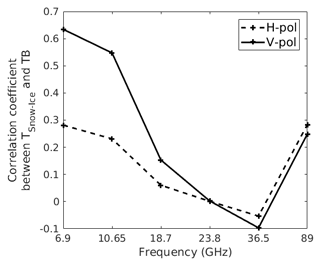Figure 5Correlation coefficient between the TSnow−Ice from IMBs and the AMSR2 TBs, as a function of AMSR2 frequency.

## 4.3 Linear regressions to retrieve the snow–ice interface temperature

To express the TSnow−Ice as a function of the TB at 6 and 10 V, the linear regressions are calculated on centred data (i.e. the anomaly). For each buoy, the averaged TSnow−Ice is subtracted from the TSnow−Ice measurements and the same is done with the TB measurements. Thus, the temperature offset between the buoys is removed and the slope of the linear regression is unchanged:

$\begin{array}{ll}& \mathrm{\Delta }{T}_{\mathrm{Snow}-\mathrm{Ice}}={a}_{\mathrm{1}}\cdot \mathrm{\Delta }{\text{TB}}_{\mathrm{6}\phantom{\rule{0.25em}{0ex}}\mathrm{or}\phantom{\rule{0.25em}{0ex}}\mathrm{10}\phantom{\rule{0.125em}{0ex}}\mathrm{V}}⇔{T}_{\mathrm{Snow}-\mathrm{Ice}}\\ \text{(3)}& & ={a}_{\mathrm{1}}\cdot {\text{TB}}_{\mathrm{6}\phantom{\rule{0.25em}{0ex}}\mathrm{or}\phantom{\rule{0.25em}{0ex}}\mathrm{10}\phantom{\rule{0.125em}{0ex}}\mathrm{V}}+{\text{offset}}_{\mathrm{buoy}},\end{array}$

with ΔTSnow−Ice and ΔTB describing the centred TSnow−Ice and TB. Figure 6 shows the linear regression between the TSnow−Ice and the TB at 6 and 10 V, using the measurements from buoys 2012G, 2012H, 2012J, and 2012L. The slope coefficients (a1) estimated between the TSnow−Ice and the TB at 6 and 10 V are 1.086±0.020 and 1.078±0.019.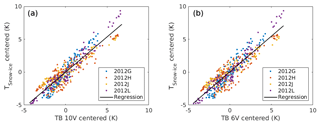Figure 6Centred TSnow−Ice expressed as a function of the centred TBs at 10 V (a) and 6 V (b). Data from the IMBs are in different colours depending on the buoy, and the linear regression is the solid black line.

The offset (offsetbuoy) in the linear regression equations between TSnow−Ice and the TB is different for each buoy, because it depends on the snow depth. The TSnow−Ice dependence on snow depth can be explained by the thermal insulation of snow . Here, we establish an empirical relationship between the TSnow−Ice corrected for the TB linear dependence at 10 or 6 V, and the snow depth as follows:

$\begin{array}{}\text{(4)}& {T}_{\mathrm{Snow}-\mathrm{Ice}}-{a}_{\mathrm{1}}\cdot {\text{TB}}_{\mathrm{10}\phantom{\rule{0.25em}{0ex}}\mathrm{or}\phantom{\rule{0.25em}{0ex}}\mathrm{6}\phantom{\rule{0.125em}{0ex}}\mathrm{V}}={a}_{\mathrm{2}}\cdot f\left({D}_{\mathrm{s}}\right)+{a}_{\mathrm{3}},\end{array}$

with f(Ds) a function of snow depth.

Three different linear regressions have been tested to relate the TSnow−Ice using the snow depth directly, the inverse of the snow depth, and the logarithm of snow depth. Figure 7 shows the TSnow−Ice corrected from TB dependence as a function of snow depth. The different regressions are tested using the training data set (IMB G, H, J, and L in 2012). The regression showing the best results uses the logarithm of the snow depth (solid black line in Fig. 7). The linear regression using the snow depth directly (dashed red line in Fig. 7) leads to an overestimation of the TSnow−Ice for large snow depth. The regression using the inverse of the snow depth (red dotted line in Fig. 7) leads to an underestimation for small snow depth. The RMSEs obtained on the TSnow−Ice are compared and the relation using the logarithm of snow depth shows the lowest RMSE. Based on these results, the final equations to relate the TSnow−Ice to the snow depth and the TB at 10 and at 6 V are as follows:

$\begin{array}{}\text{(5)}& {T}_{\mathrm{Snow}-\mathrm{Ice}}=\mathrm{1.078}\cdot {\text{TB}}_{\mathrm{10}\phantom{\rule{0.125em}{0ex}}\mathrm{V}}+\mathrm{5.67}\cdot \mathrm{log}\left({D}_{\mathrm{s}}\right)-\mathrm{5.13}\end{array}$

$\begin{array}{}\text{(6)}& {T}_{\mathrm{Snow}-\mathrm{Ice}}=\mathrm{1.086}\cdot {\text{TB}}_{\mathrm{6}\phantom{\rule{0.125em}{0ex}}\mathrm{V}}+\mathrm{3.98}\cdot \mathrm{log}\left({D}_{\mathrm{s}}\right)-\mathrm{10.70},\end{array}$

where TSnow−Ice and TB are expressed in kelvin, and Ds is expressed in metres.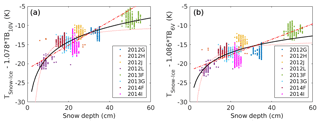Figure 7TSnow−Ice corrected for the 10 V TB (a) and of the 6 V TB (b) dependence as a function of snow depth. Data from the IMBs are represented by different colours, the regression using the snow depth is shown by the dashed red line, the regression using the inverse of snow depth by the dotted red line, and the regression using the logarithm of the snow depth by the solid black line.

## 4.4 Results of the snow–ice interface temperature retrieval

Figure 8 shows the comparisons between the observed TSnow−Ice and the regressed TSnow−Ice using the 10 and 6 V TBs (Eqs. 5 and 6), and the in situ snow depth measured by the acoustic sounder of IMB. The RMSEs are computed using the IMB 2013F, 2013G, 2014F, and 2014I. The regression of the TSnow−Ice using the in situ snow depth with the 10 V TBs (Eq. 5) is slightly better (RMSE =1.78 K) than the regression with the 6 V TBs (Eq. 6) (RMSE =1.98 K). The variability due to the snow depth is better described with the regression using the 10 V TBs. Figure 9 is the same as Fig. 8 but with our snow depth estimation (Eq. 2). The RMSEs are 2.87 K for the 10 V regression and 2.90 K for the 6 V regression. The results are degraded because of the snow depth regression, especially for the buoys with thick snow (∼50 cm) or thin snow (∼5 cm) (e.g. buoy 2013F and buoy 2012L). Note that the regression is tested with IMBs, which are all located on MYI. However, using our algorithm to derive the TSnow−Ice is also applicable over FYI areas, as our snow depth algorithm is applicable to both ice types and our TSnow−Ice algorithm uses the channels 10 or 6 V, which have limited sensitivity to the ice type .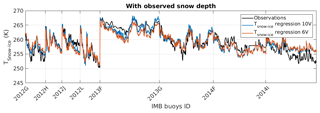Figure 8Time series of the comparisons between TSnow−Ice observations from IMBs (black line), and TSnow−Ice regressions with TBs at 10 V (blue line) and at 6 V (red line). The snow depth used in Eqs. (5) and (6) is the snow depth observed by the IMB sounder. The beginning of the measurements with a new IMB is indicated on the x axis.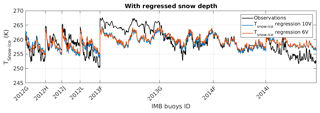Figure 9Same as Fig.  8, using the regressed snow depth (Eq. 2) in place of in situ snow depth

5 Sea ice effective temperature estimation

## 5.1 Bias between the model and the observations

Teff is related to the frequency and the incidence angle of the satellite observations. It is not a geophysical variable that we can measure directly as an in situ parameter. A microwave emission model has to be used to computed the Teffs from the geophysical parameters. The Teff used here is available from a simulated data set using a thermodynamical model and the microwave emission model, MEMLS. The model set-up and the simulations are described in . In this data set, the TBs and the Teffs are simulated using the TSnow−Ice and the input snow and ice profiles from the thermodynamical model. Even though the simulated TB data are comparable to observations in terms of mean and standard deviation, both the thermodynamical model and the emission model are based on physical equations and are not tuned to observations. TBs simulated with MEMLS are not fitted to AMSR2 TBs, meaning that a bias is expected between the TSnow−Ice of the MEMLS-simulated data set (TSnow-Ice MEMLS) and the TSnow−Ice estimated with our regression.

The bias obtained is the mean value of the difference between the TSnow-Ice MEMLS, and the TSnow−Ice regressed from Eqs. (5) and (6) using the TBs of the MEMLS-simulated data set as inputs. Biases of 3.97 and 4.01 K are estimated for the regressions with 10 and 6 V respectively. The RMSEs computed between the TSnow-Ice MEMLS and the TSnow−Ice regressed and corrected for the biases at 10 and 6 V are 2.7 and 2.07 K.

Figure 10 shows the TSnow−Ice from the MEMLS-simulated data set as a function of TB at 10 and 6 V, and the TSnow−Ice computed from our regressions (Eqs. 5 and 6), with and without the bias correction. We can see that the slopes of our linear regressions are consistent with the data simulated from MEMLS.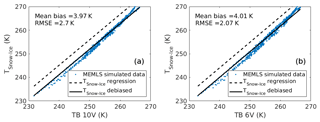Figure 10Comparisons between the TSnow-Ice MEMLS from the MEMLS-simulated data is shown with blue points, the regressed TSnow−Ice (Eqs. 5 and 6) with a dashed black line, and the regressed TSnow−Ice debiased to fit the MEMLS simulations with a solid black line at 10 V (a) and 6 V (b) channels.

## 5.2 Linear regression between the effective temperature and the snow–ice interface temperature

The Teff near 50 GHz in vertical polarization is correlated with the TSnow−Ice and it can be expressed as a linear function of the TSnow−Ice:

$\begin{array}{}\text{(7)}& {T}_{\mathrm{eff}\left(\mathrm{freq},\phantom{\rule{0.125em}{0ex}}\mathrm{pol}\right)}={b}_{\mathrm{1}\left(\mathrm{freq},\phantom{\rule{0.125em}{0ex}}\mathrm{pol}\right)}\cdot {T}_{\mathrm{Snow}\text{-}\mathrm{Ice}\phantom{\rule{0.25em}{0ex}}\mathrm{MEMLS}}+{b}_{\mathrm{2}\left(\mathrm{freq},\phantom{\rule{0.125em}{0ex}}\mathrm{pol}\right)},\end{array}$

with Teff, b1, and b2 depending on the frequency (freq) and on the polarization (pol). We use the MEMLS-simulated data set to calculate the linear regression between the TSnow−Ice and the Teff at 6.9, 10.65, 18.7, 23.8, 36.5, 50, and 89 GHz in vertical polarization. Teffs at vertical and horizontal polarizations are about the same. Only the vertical polarization is considered here, because TBs measurements are noisier at horizontal polarization due to the variability of sea ice emissivity at this polarization.

Figure 11 shows the Teff at 50 V as a function of TSnow−Ice. The linear regressions between the TSnow−Ice and the Teff at different frequencies are computed. The coefficients b1 and b2 of Eq. (7) are given in Table 2. The slope coefficient of the regression increases with frequency, meaning that the sensitivity of the Teff to the TSnow−Ice is increasing with frequency between 6 and 89 GHz. A slope coefficient lower than 1 means that the penetration depth at the given frequency is deeper than snow–ice interface. At 50 GHz the slope coefficient is near to 1, meaning that the penetration depth is close to the depth of the snow–ice interface. The RMSEs are below 1 K, with the regression of Teff at 50 V showing the lowest RMSE (0.33 K), and at 89 V showing the highest RMSE (0.92 K).

Table 2Regressions of the Teff for different frequencies at vertical polarization as a function of the TSnow−Ice (see Eq. 7) using the MEMLS-simulated data set.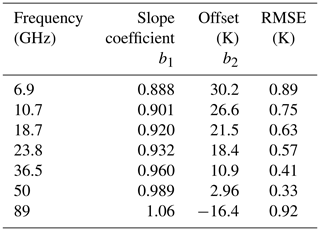These linear regressions between the Teff and the TSnow-Ice MEMLS (Eq. 7) are the final step in retrieving the Teff of sea ice at microwave frequencies as a function of TBs, using the work in the previous sections to express the TSnow−Ice as a function of TBs (Eqs. 2, and 5 or 6). The biases between the AMSR2 observations and the MEMLS-simulated data set are taken into account, replacing TSnow-Ice MEMLS by TSnow−Ice estimated from AMSR2 TBs with a bias correction (see Table 2):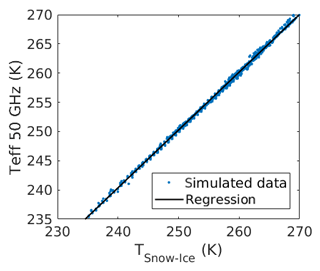Figure 11Regression of the Teff as a function of TSnow−Ice at 50 GHz in vertical polarization. The data from the MEMLS simulations are in blue points and the linear regression is the solid black line.

6 Discussion

For days in November, January, and April, Fig. 12 shows the maps of the snow depth estimated with our multilinear regression (Eq. 2), the TSnow−Ice estimated with our multilinear regression (Eq. 5), and the MYI concentration products from the University of Bremen (https://seaice.uni-bremen.de, last access: 1 November 2018). Maps of the MYI concentration from University of Bremen are derived from AMSR2 and from the Advanced SCATterometer (ASCAT) with the method of . To perform our regressions, we use the AMSR2 TBs (Level L1R) provided by JAXA and the SIC from the European Centre for Medium-Range Weather Forecasts (ECMWF) Reanalysis Interim (ERA-Interim) data. Only the areas with 100 % SIC are considered to compute the snow depth on sea ice and the TSnow−Ice with our method.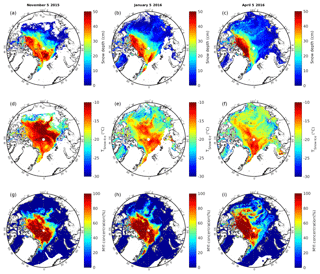Figure 12Maps of the snow depth (a, b, c) and the TSnow−Ice (d, e, f) estimated from our multilinear regression using AMSR2 TBs, with multi-year ice (MYI) concentration products (g, h, i) from the University of Bremen on 5 November 2015 (a, d, g), 5 January 2016 (b, e, h), and 5 April 2016 (c, f, i).

The results show that the snow depth is larger (40 cm) in the north of Greenland due to the presence of drift snow caused by the numerous pressure ridges present in this area (Hanson1980), as anticipated. We can observe that the snow depth is larger in areas with larger MYI concentrations. The variability of the snow cover is low during winter, as the snow depth reaches a maximum by December and remains relatively unchanged until snowmelt .

For TSnow−Ice, in January and April when the air temperature is cold (between −20 and −30C over the whole Arctic, on 5 January and 5 April 2016 from ERA-Interim air temperature), the areas with large snow depth show larger TSnow−Ice because of the thermal insulation power of the snow. It is different in November: the air temperature is warmer (−5C near Kara Sea, −15C near Laptev Sea, and −25C in the central Arctic and Beaufort seas, on 5 November 2015 from ERA-Interim air temperature) and the areas with thinner snow show larger TSnow−Ice which are close to the air temperature . Note that we can observe low TSnow−Ice in some locations near the sea ice margins due to the presence of open ocean in the satellite footprint. As the brightness temperature of open water is low, the total brightness temperature measured is decreased and it impacts our TSnow−Ice estimation.

Visually the TSnow−Ice shows a high correlation with the distribution patterns of multiyear ice concentration on the same days: the highest values are found in the north of Greenland and in the Canada Basin, with some branches of higher values extending from there towards the Siberian coast, marking the Beaufort Gyre of the Arctic sea ice drift (see the animations for the same year at https://seaice.uni-bremen.de/multiyear-ice-concentration/animations/, last access: 1 November 2018). The main differences between FYI and MYI are, on average, the higher thickness of MYI and its higher snow load. Both effects will influence the TSnow−Ice. Under the same conditions, a higher ice thickness will lead to a lower TSnow−Ice. In contrast, it will be higher if only the snow depth is increased. The positive correlation between MYI concentration and TSnow−Ice suggests that the influence of the higher snow depth on MYI outbalances that of the higher ice thickness on the TSnow−Ice, emphasizing the important role of snow on sea ice in its thermodynamic balance.

The similar patterns observed between the maps of the TSnow−Ice and the MYI concentration on Fig. 12 are encouraging and give confidence in the methodology developed here, as these MYI concentration products are from independent work done at the University of Bremen and distributed daily to users. However it should be noted that the input channels of both methods overlap in some AMSR2 channels, and even different channels show some covariance .

7 Conclusions

We derive simple algorithms to estimate sea ice parameters such as the snow depth, the TSnow−Ice, and the Teff of sea ice at microwave frequencies, from AMSR2 channels. This is achieved using the ESA RRDP, which contains AMSR2 data collocated with IMB data and OIB campaign data. In addition, simulated TB outputs from a sea ice version of MEMLS are used for the regression of the Teff. All the equations used to retrieve these sea ice parameters are derived using several linear and multilinear regressions.

Our regression to retrieve the snow depth over winter Arctic sea ice uses the TBs at 6.9, 18.7, and 36.5 GHz in vertical polarization. A RMSE of 5.1 cm is obtained between the estimated and the IMB snow depths using an independent IMB test data set. This snow depth retrieval is applicable to FYI and MYI, with lower uncertainties for FYI than for MYI (3.9 cm compared to 7.2 cm). To retrieve the TSnow−Ice, two relations are derived using two different AMSR2 channels (10 or 6 V) and the estimated snow depth. The two regressions show similar results. The errors are 2.87 and 2.90 K at 10 and 6 V. This TSnow−Ice retrieval has been tested only for MYI. It can also be applied to FYI, as the 6 and 10 V channels have limited sensitivity to the ice type . Finally the Teffs at 6.9, 10.65, 18.7, 23.8, 36.5, 50, and 89 GHz in vertical polarization are retrieved as a function of TSnow−Ice using linear regressions. At the final step, the RMSEs of the linear regressions between the simulated TSnow−Ice and the Teff for all channels are lower than 1 K, with a minimum value of 0.33 K at 50 GHz, which is a key frequency for atmosphere temperature retrieval. The methodology used to estimate snow depth and TSnow−Ice has been applied to several days during winter. It shows consistent results with MYI concentration estimates obtained independently.

These algorithms can be used to create snow depth and TSnow−Ice products which can improve the study of sea ice variability (e.g. sea ice growth). Information on the TSnow−Ice may help in sea ice models by constraining the sea ice temperature gradient and the thermodynamical ice growth. The Teff estimations can be used in atmospheric radiative transfer calculations and to reduce noise in SIC retrieval algorithms (e.g. EUMETSAT OSISAF global SIC product).

Data availability

The round robin data package used for this study is publicly accessible at https://figshare.com/articles/Reference_dataset_for_sea_ice_concentration/6626549 .

Author contributions

This study was conducted by LK and supervised by RTT and CP. GH contributed to the analysis and to the correction of the draft.

Competing interests

The authors declare that they have no conflict of interest.

Acknowledgements

This research was funded by EUMETSAT OSISAF (OSI VS17 03) and the PNTS (Programme national de télédédtection spatiale). The authors acknowledge the support from the EUMETSAT OSISAF visiting scientist programme and the Danish Meteorological Institute for its welcome. We also acknowledge the reviewers for their precious comments, which improved this manuscript a lot.

Review statement

This paper was edited by John Yackel and reviewed by Leif Toudal Pedersen and one anonymous referee.

References

Baordo, F. and Geer, A.: Microwave Surface Emissivity over sea-ice, EUMETSAF NWP SAF, Tech. Rep. NWPSAF_EC_VS_026, 1–30, 2015. a

Comiso, J.: Sea ice effective microwave emissivities from satellite passive microwave and infrared observations, J. Geophys. Res., 88, 7686–7704, 1983. a, b, c

Comiso, J., Cavalieri, D., and Markus, T.: Sea ice concentration, ice temperature, and snow depth using AMSR-E data, IEEE T. Geosci. Remote, 41, 243–252, 2003. a

Draper, N. R. and Smith, H.: Applied regression analysis, John Wiley & Sons, Inc., Hoboken, NJ, USA, 1998. a

Dybkjær, G., Tonboe, R., and Høyer, J. L.: Arctic surface temperatures from Metop AVHRR compared to in situ ocean and land data, Ocean Sci., 8, 959–970, https://doi.org/10.5194/os-8-959-2012, 2012. a

English, S. J.: The Importance of Accurate Skin Temperature in Assimilating Radiances From Satellite Sounding Instruments, IEEE T. Geosci. Remote, 46, 403–408, 2008. a

Fichefet, T. and Maqueda, M. A. M.: Modelling the influence of snow accumulation and snow-ice formation on the seasonal cycle of the Antarctic sea-ice cover, Clim. Dynam., 15, 251–268, 1999. a

Grönfeldt, I.: Snow and sea ice temperature profiles from satellite data and ice mass balance buoys, Lund University, Sweden, Tech. rep., 370, 1–72, 2015. a

Hall, A.: The role of surface albedo feedback in climate, J. Climate, 17, 1550–1568, 2004. a

Hanson, A. M.: The Snow Cover of Sea Ice during the Arctic Ice Dynamics Joint Experiment, 1975 to 1976, Arctic Alpine Res., 12, 215–226, https://doi.org/10.1080/00040851.1980.12004180, 1980. a

Harlow, R.: Millimeter Microwave Emissivities and Effective Temperatures of Snow-Covered Surfaces: Evidence for Lambertian Surface Scattering, IEEE T. Geosci. Remote, 47, 1957–1970, 2009. a

Harlow, R. C.: Sea Ice Emissivities and Effective Temperatures at MHS Frequencies: An Analysis of Airborne Microwave Data Measured During Two Arctic Campaigns, IEEE T. Geosci. Remote, 49, 1223–1237, 2011. a

Holt, B., Johnson, M. P., Perkovic-Martin, D., and Panzer, B.: Snow depth on Arctic sea ice derived from radar: In situ comparisons and time series analysis, J. Geophys. Res.-Oceans, 120, 4260–4287, 2015. a

Kern, S. and Ozsoy-Çiçek, B.: Satellite remote sensing of snow depth on Antarctic Sea Ice: An inter-comparison of two empirical approaches, Remote Sensing, 8, 450, https://doi.org/10.3390/rs8060450, 2016. a

Kurtz, N. T., Farrell, S. L., Studinger, M., Galin, N., Harbeck, J. P., Lindsay, R., Onana, V. D., Panzer, B., and Sonntag, J. G.: Sea ice thickness, freeboard, and snow depth products from Operation IceBridge airborne data, The Cryosphere, 7, 1035–1056, https://doi.org/10.5194/tc-7-1035-2013, 2013. a

Kwok, R. and Maksym, T.: Snow depth of the Weddell and Bellingshausen sea ice covers from IceBridge surveys in 2010 and 2011: An examination, J. Geophys. Res.-Oceans, 119, 4141–4167, 2014. a, b

Kwok, R., Kurtz, N. T., Brucker, L., Ivanoff, A., Newman, T., Farrell, S. L., King, J., Howell, S., Webster, M. A., Paden, J., Leuschen, C., MacGregor, J. A., Richter-Menge, J., Harbeck, J., and Tschudi, M.: Intercomparison of snow depth retrievals over Arctic sea ice from radar data acquired by Operation IceBridge, The Cryosphere, 11, 2571–2593, https://doi.org/10.5194/tc-11-2571-2017, 2017. a

Lecomte, O., Fichefet, T., Vancoppenolle, M., and Nicolaus, M.: A new snow thermodynamic scheme for large-scale sea-ice models, Ann. Glaciol., 52, 337–346, https://doi.org/10.3189/172756411795931453, 2011. a

Maaß, N., Kaleschke, L., Tian-Kunze, X., and Drusch, M.: Snow thickness retrieval over thick Arctic sea ice using SMOS satellite data, The Cryosphere, 7, 1971–1989, https://doi.org/10.5194/tc-7-1971-2013, 2013. a

Maeda, T., Imaoka, K., Kachi, M., Fujii, H., Shibata, A., Naoki, K., Kasahara, M., Ito, N., Nakagawa, K., and Oki, T.: Status of GCOM-W1/AMSR2 development, algorithms, and products, in: Sensors, Systems, and Next-Generation Satellites XV, SPIE Remote Sensing, 2011, Prague, Czech Republic, edited by: Meynart, R., Neeck, S. P., and Shimoda, H., SPIE, 8176, https://doi.org/10.1117/12.898381, 2011. a

Maeda, T., Taniguchi, Y., and Imaoka, K.: GCOM-W1 AMSR2 level 1R product: Dataset of brightness temperature modified using the antenna pattern matching technique, IEEE T. Geosci. Remote, 54, 770–782, 2016. a

Markus, T. and Cavalieri, D. J.: Snow Depth Distribution Over Sea Ice in the Southern Ocean from Satellite Passive Microwave Data, in: Antarctic Sea Ice: Physical Processes, Interactions and Variability, edited by: Jeffries, M. O., American Geophysical Union, Washington, DC, 19–39, 1998. a, b

Markus, T., Massom, R., Worby, A., Lytle, V., Kurtz, N., and Maksym, T.: Freeboard, snow depth and sea-ice roughness in East Antarctica from in situ and multiple satellite data, Ann. Glaciol., 52, 242–248, 2011. a

Mathew, N., Heygster, G., Melsheimer, C., and Kaleschke, L.: Surface Emissivity of Arctic Sea Ice at AMSU Window Frequencies, IEEE T. Geosci. Remote, 46, 2298–2306, 2008. a

Mätzler, C.: Thermal microwave radiation applications for remote sensing, Institution of Engineering and Technology, London, UK, 2006. a

Maykut, G. A. and Untersteiner, N.: Some results from a time-dependent thermodynamic model of sea ice, J. Geophys. Res., 76, 1550–1575, https://doi.org/10.1029/JC076i006p01550, 1971. a

Pedersen, L. F. and Saldo, R.: Sea Ice Concentration (SIC) Round Robin Data Package, Sea Ice Climate Initiative: Phase 2, ESA, Tech. Rep. SICCI-RRDP-07-16 Version: 1.4, 2016. a

Pedersen, L. T., Saldo, R., Ivanova, N., Kern, S., Heygster, G., Tonboe, R., Huntemann, M., Ozsoy, B., Ardhuin, F., and Kaleschke, L.: Rasmus Reference dataset for sea ice concentration, Fileset, figshare, https://doi.org/10.6084/m9.figshare.6626549.v3, 2018. a, b, c, d

Perovich, D. and Richter-Menge, J. A.: From points to Poles: extrapolating point measurements of sea-ice mass balance, Ann. Glaciol., 44, 188–192, 2006. a

Perovich, D. K. and Elder, B. C.: Temporal evolution of Arctic sea-ice temperature, Ann. Glaciol., 33, 207–211, 2001. a

Perovich, D., Richter-Menge, J., and Polashenski, C.: Observing and understanding climate change: Monitoring the mass balance, motion, and thickness of Arctic sea ice, available at: http://imb-crrel-dartmouth.org, last access: 17 April 2019. a

Richter-Menge, J. A., Perovich, D. K., Elder, B. C., Claffey, K., Rigor, I., and Ortmeyer, M.: Ice mass-balance buoys: a tool for measuring and attributing changes in the thickness of the Arctic sea-ice cover, Ann. Glaciol., 44, 205–210, 2006. a

Rosenkranz, P. W. and Mätzler, C.: Dependence of AMSU-A Brightness Temperatures on Scattering From Antarctic Firn and Correlation With Polarization of SSM/I Data, IEEE Geosci. Remote S., 5, 769–773, 2008. a

Rostosky, P., Spreen, G., Farrell, S. L., Frost, T., Heygster, G., and Melsheimer, C.: Snow Depth Retrieval on Arctic Sea Ice From Passive Microwave Radiometers–Improvements and Extensions to Multiyear Ice Using Lower Frequencies, J. Geophys. Res.-Oceans, 123, 7120–7138, 2018. a

Sato, K. and Inoue, J.: Comparison of Arctic sea ice thickness and snow depth estimates from CFSR with in situ observations, Clim. Dynam., 50, 289–301, 2018. a, b

Scarlat, R. C., Heygster, G., and Pedersen, L. T.: Experiences with an Optimal estimation algorithm for surface and atmospheric parameter retrieval from passive microwave data in the arctic, IEEE J. Sel. Top. Appl., 10, 3934–3947, 2017. a

Shalina, E. V. and Sandven, S.: Snow depth on Arctic sea ice from historical in situ data, The Cryosphere, 12, 1867–1886, https://doi.org/10.5194/tc-12-1867-2018, 2018. a

Spreen, G., Kaleschke, L., and Heygster, G.: Sea ice remote sensing using AMSR-E 89-GHz channels, J. Geophys. Res., 113, C02S03, https://doi.org/10.1029/2005JC003384, 2008. a, b, c

Sturm, M., Holmgren, J., and Perovich, D. K.: Winter snow cover on the sea ice of the Arctic Ocean at the Surface Heat Budget of the Arctic Ocean (SHEBA): Temporal evolution and spatial variability, J. Geophys. Res., 107, 8047, https://doi.org/10.1029/2000JC000400, 2002. a

Tonboe, R. T.: The simulated sea ice thermal microwave emission at window and sounding frequencies, Tellus A, 62, 333–344, 2010. a, b, c, d

Tonboe, R. T., Dybkjær, G., and Høyer, J. L.: Simulations of the snow covered sea ice surface temperature and microwave effective temperature, Tellus A, 63, 1028–1037, 2011. a, b, c, d

Tonboe, R. T., Schyberg, H., Nielsen, E., Rune Larsen, K., and Tveter, F. T.: The EUMETSAT OSI SAF near 50 GHz sea ice emissivity model, Tellus A, 65, 18380, https://doi.org/10.3402/tellusa.v65i0.18380, 2013. a, b

Untersteiner, N.: The geophysics of sea ice, Springer, Boston, Massachusetts, 1986. a

Wang, D., Prigent, C., Kilic, L., Fox, S., Harlow, C., Jimenez, C., Aires, F., Grassotti, C., and Karbou, F.: Surface Emissivity at Microwaves to Millimeter Waves over Polar Regions: Parameterization and Evaluation with Aircraft Experiments, J. Atmos. Ocean. Tech., 34, 1039–1059, https://doi.org/10.1175/JTECH-D-16-0188.1, 2017. a

Warren, S. G., Rigor, I. G., Untersteiner, N., Radionov, V. F., Bryazgin, N. N., Aleksandrov, Y. I., and Colony, R.: Snow Depth on Arctic Sea Ice, J. Climate, 12, 1814–1829, 1999. a

Wiesmann, A. and Mätzler, C.: Microwave Emission Model of Layered Snowpacks, Remote Sens. Environ., 70, 307–316, 1999.  a

Ye, Y., Heygster, G., and Shokr, M.: Improving Multiyear Ice Concentration Estimates With Reanalysis Air Temperatures, IEEE T. Geosci. Remote, 54, 2602–2614, 2016a. a

Ye, Y., Shokr, M., Heygster, G., and Spreen, G.: Improving multiyear sea ice concentration estimates with sea ice drift, Remote Sensing, 8, 397, https://doi.org/10.3390/rs8050397, 2016b. a